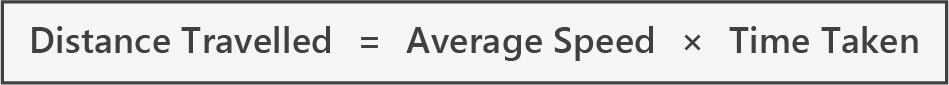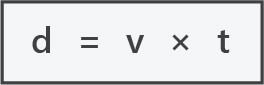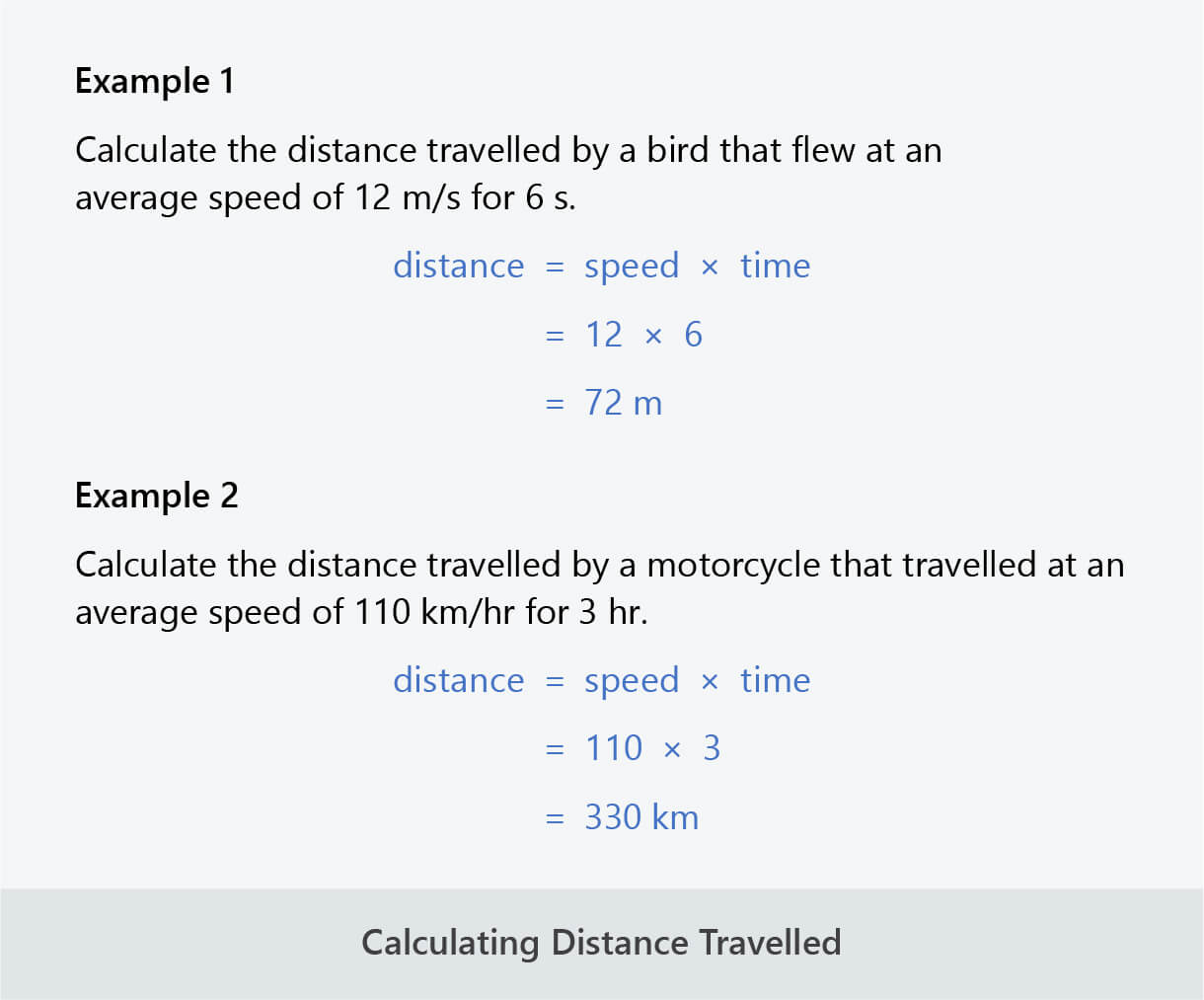# 5 | Calculating Distance Travelled

## Calculating Distance

• The formula for average speed can be rearranged to calculate distance travelled:• This can be abbreviated as:• The units for distance will depend on the units for average speed.
• If speed is measured in metres per second (m/s), the units for distance will be metres (m).
• If speed is measured in kilometres per hour (km/hr), the units for distance will be kilometres (km).• The units for speed and time need to correspond with each other for the formula to work. Therefore, it is sometimes necessary to convert the units given for time before entering the value into the formula.
• For example, if you are given speed in km/hr and time in min, convert time from min to hr by dividing by 60.